# Lightfield Camera Simulation

## ( This is not the final report ! )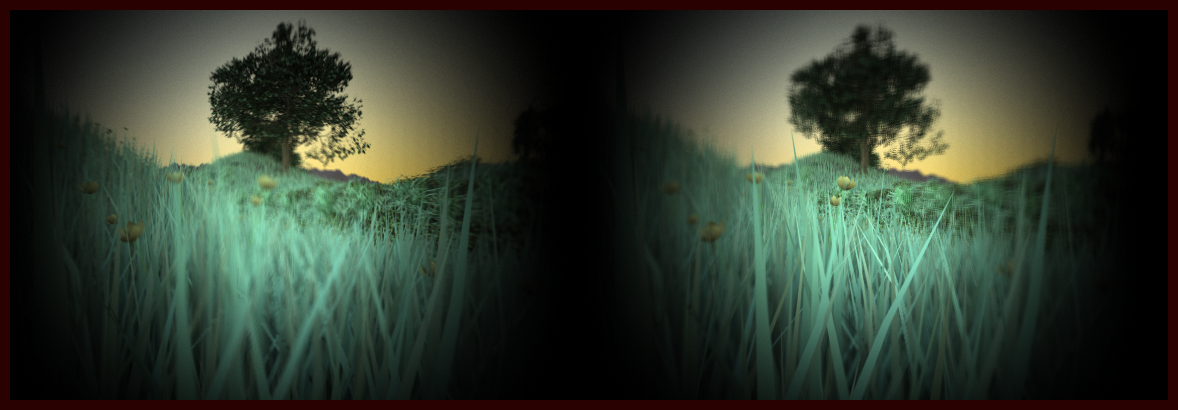Fig 1. The final submission image (captured with a single element spherical lens) that demonstrates after the fact refocusing, spherical distortion and vignetting from lightfield imaging. The left panel is focused at the far tree while the right image is focused on the near flower. Note how the grass blades near the edge of the vignette, due to spherical lens distortion, are not focused at the same time as the flower though they belong to the same $z$ plane.
 Video demonstrating after the fact refocusing, multiple view point rendering and vignetting effects of lightfield imaging. Note that the multi viewpoint scenes (when the camera seems to pan around) are noisier than refocused images.

In this project we simulated a plenoptic camera system similar to Lytro within PBRT. For this we followed the recipe of Ng et.al  and extended the realistic camera system of Kolb et.al  by inserting a microlens array between the film plane and the main lens (see Figure 2). A microlens is vanishingly small compared to the main lens and therefore we applied simple pinhole model to streamline our implementation. However, replacing a pinhole with a real miniature lens should be straightforward. Our main aim was to implement after the fact refocusing algorithm, multiple viewpoint rendering while focusing on different intriguing use cases that are unique for such lightfield imaging.

## Lightfield Data

A lightfield is a 4d function $(s,t,u,v)$ where $(s,t)$ are the spatial dimensions and $(u,v)$ are the angular. For practical purposes this 4d function is often sampled on a 2d image as shown in Figure 3. The lenslet array design of Ng et.al  -- which we adopted for our project -- captures the raw lightfield data in the lenslet format (Figure 2a). Now, pixels with the same $(u,v)$ coordinate under every lenslet image (the red circles) can be aggregated to form a subaperture image (the green box in Figure 3b) that has the same resolution as the number of microlens in each dimension. This image is equivalent to taking a pinhole image from one of the locations on the main lens. Therefore, a lightfield data can also be stored in the subaperture format as shown in Figure 3b, where the subaperture images are slightly shifted from each other due to parallax. Here we would like to point out that this is essentially the same format of data that PiCam  of Pelican Imaging captures.

## Refocusing

In this project we were only interested in computing a synthetic focus plane $s'$ while keeping the main lens $u$ and microlens plane $s$ intact as illustrated in Figure 4. Now, in order to shade a synthetic pixel at location $s'$ we need to sample the captured lightfield by tracing rays from every point $u$ on the main lens that passes through $s'$. Therefore, the first step is to compute the intersection $s$ given $s'$, $u$ and $\alpha$ - which is the distance of the synthetic plane from the main lens as a fraction of the sensor distance $F$. This intersection, after simplifying equations of Ng et.al , is given by: $$s = \frac{1}{\alpha} \left( s' - (1-\alpha) u \right)$$ Once this intersection of $s$ is established, the irradiance of a synthetic pixel $E(s',t')$ is given by the following integral -- where $L(u,v,s,t)$ is the captured light field: $$E(s',t') = \int_{u,v} L( u,v, \frac{1}{\alpha} \left( s' - (1-\alpha) u \right), \frac{1}{\alpha} \left( t' - (1-\alpha) v \right) dudv$$Fig 4. Synthetic refocusing is performed at an arbitrary plane $s'$ by tracing rays, through a given synthetic pixel, that pass through the subaperture spanned by $u$ from the main lens to the captured lightfield plane $s$. The distance of the synthetic plane from the main lens is parameterized by $\alpha$ which is the fraction of total distance from the main lens to the microlens array.
Even though the above refocusing integral is only 2d, we still applied monte-carlo technique to mask off subsampling aliasing with random noise, which is visually less objectionable.

We took off the $1/\alpha$ term from the above refocusing equation to get rid of the scaling effect from our synthetically refocused image. This is equivalent to sampling the synthetic plane more densely as we move it closer to the main lens thus keeping the dimensions of the rendered image (in pixels) the same. We eventually used the following modified integral to compute our final refocused image $$E(s',t') = \int_{u,v} L( u,v, \left( s' - (1-\alpha) u \right), \left( t' - (1-\alpha) v \right) dudv$$

Since the intersection of $s,t$ and the integrand $u,v$ are all continuous variables we performed interpolation on the discrete lightfield $L(u,v,s,t)$ to convert it into a continuous function. For this we used three different interpolation techniques; i) Nearest Neighbor (NN) in all 4 dimensions, ii) Linear interpolation in $(s,t)$ but NN in $(u,v)$ and iii) Linear interpolation in all 4 dimensions. We noticed a greater reduction of artifacts as we linearly interpolated along $(s,t)$ dimensions alone. Figure 5 shows the effect of these interpolation techniques.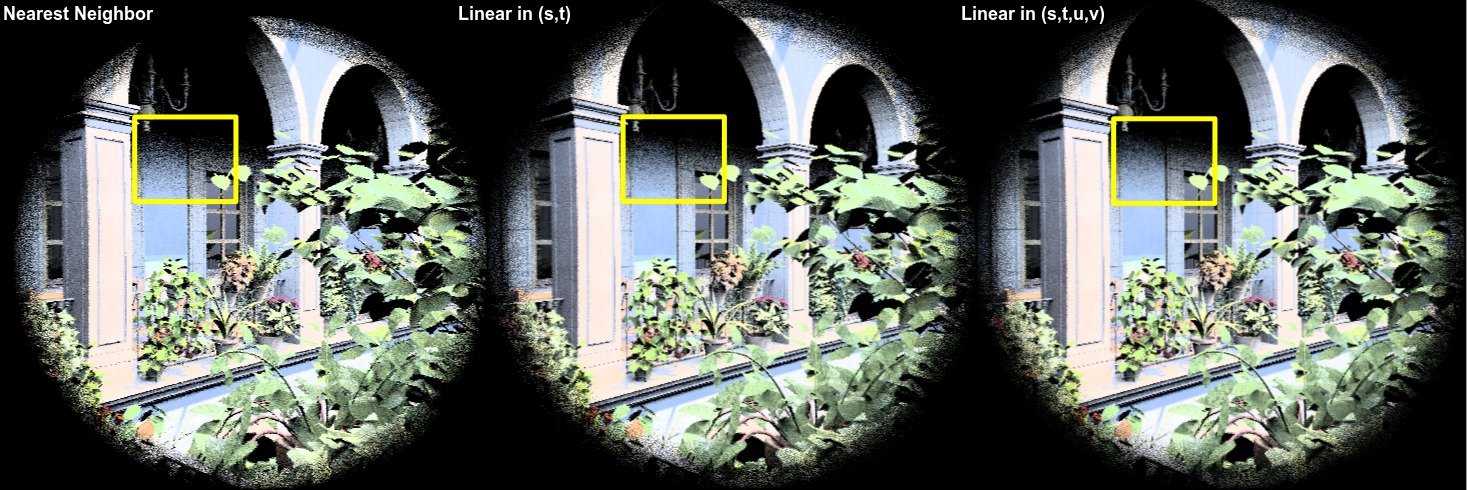Fig 5. Effect of different interpolation techniques. Linear interpolation along $(s,t)$ alone seemed to have more significant effect than $(u,v)$

## Results

### Refocusing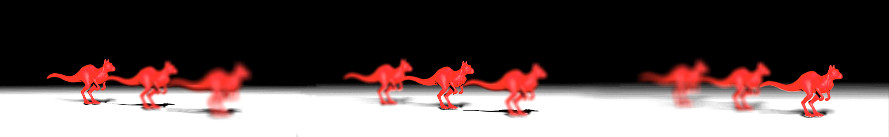Fig 6. Test scene to demonstrate refocusing algorithm. One killeroo is brought to focus at a time.
In Figure 6 we setup a simple scene with three killeroos separated along the $z$ axis which was then ray traced to capture a lightfield with a spatial resolution of $(800\times 600)$ and an angular resolution of $(32\times 32)$. This produces a raw lightfield image data of size $(25600\times 19200)$. We then refocused on a single killeroo at a time demonstrating the ability of the lightfield to refocus after the fact.

### Playing with depth map

The synthetic focus plane being virtual we were able to make the $\alpha$ parameter vary over the focus plane thus simulating many interesting imaging phenomena, e.g. tilt-shift (see Figure 7) to generate an all-in-focus image, and selective focusing (see Figure 8) by hand drawing depth maps.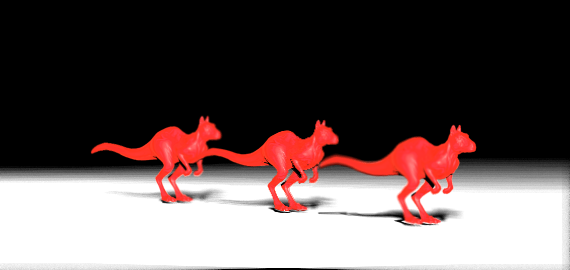Fig 7. Synthetic tilt shift imaging to produce an all-in-focus image. The left image is a hand drawn depth map that encodes varying $\alpha$ over the synthetic focus plane. The right image is the refocused image that has all the three killeroos focused at the same time.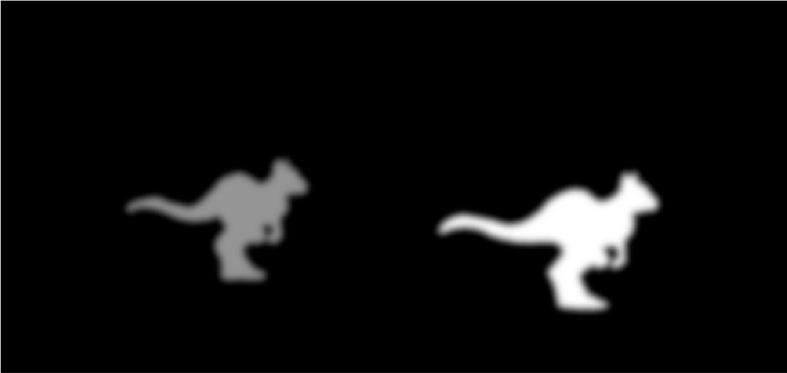Fig 8. Selective focusing by hand drawing depth map. The left image is the depth map and the right image is a resultant refocused image where the farthest and the nearest killeroos are in focus while the middle one is not. This is unattainable in a conventional camera.

### Impossible Macro Photography

 Video demonstrating objects placed closer than one focal distance can still be brought to focus with lightfield imaging.
A subject placed closer than one focal distance of the main lens cannot be brought to focus by conventional camera but the above video demonstrates that this can be achieved with a lightfield camera. For this we placed a textured ball closer than the focal distance of the main lens and captured a lightfield. Next we applied our refocusing algorithm naively which was already able to focus the ball thus achieving a level of macro photography that is not possible with a conventional camera.

## Extra Scene and Spherical Aberration

Here we captured lightfield using the stock San Miguel scene (sanmiguel_cam20.pbrt) that has a lot of depth complexity. The following video demonstrates the same refocusing and multiple viewpoint rendering as well as Spherical Aberration due to a single spherical lens element. Note how the leaves along the edge of the vignette are brought to focus earlier than the ones in the center of the scene even though they reside approximately on the same $z$ plane. This is because light rays that pass through the edge of a spherical lens converge earlier than the focal distance. It is also interesting to note how the subaperture images, while rendering multi viewpoint shots, tend to be noisier than the refocused images. This is because less light rays are integrated during subaperture image reconstruction.
 Refocusing and multiple viewpoint rendering with the stock San-Miguel scene (sanmiguel_cam20.pbrt). For this we used a single element spherical lens that suffers from spherical aberration. The leaves along the vignette edge are focused earlier then the ones in the middle, though they are approximately on the same $z$ plane, as we move our focus closer to the nearer planes. Also note, how the subaperture images while generating multi viewpoint scenes are noiser than the full aperture refocused image.

## Final Remarks

We found lightfield imaging to be an interesting method for photography as one never has to worry about setting up a capture but simply capture the precious moment that may not last more than a split second. One of the challenges in this space is the reduced resolution of the refocused image due to fitting the angular information into the same image sensor real estate. However, lots of work along synthesizing super-resolution image from a lightfield are still in progress [3,4], and it has already been shown that the information in a lightfield is very rich and the final refocused image resolution does not always necessarily gets divided by the angular resolution.

## References

 Kolb, Craig, Don Mitchell, and Pat Hanrahan. "A realistic camera model for computer graphics." Proceedings of the 22nd annual conference on Computer graphics and interactive techniques. ACM, 1995.

 Ng, Ren, et al. "Light field photography with a hand-held plenoptic camera." Computer Science Technical Report CSTR 2.11 (2005).

 Venkataraman, Kartik, et al. "PiCam: an ultra-thin high performance monolithic camera array." ACM Transactions on Graphics (TOG) 32.6 (2013): 166.

 Bishop, Tom E., Sara Zanetti, and Paolo Favaro. "Light field superresolution." Computational Photography (ICCP), 2009 IEEE International Conference on. IEEE, 2009.